# Spatial data in R: Using R as a GIS

A tutorial to perform basic operations with spatial data in R, such as importing and exporting data (both vectorial and raster), plotting, analysing and making maps.

v 2.1

18-12-2013

# 1. INTRODUCTION

R is great not only for doing statistics, but also for many other tasks, including GIS analysis and working with spatial data. For instance, R is capable of doing wonderful maps such as this or this. In this tutorial I will show some basic GIS functionality in R.

#### Basic packages

``````
library(sp)  # classes for spatial data
library(raster)  # grids, rasters
library(rasterVis)  # raster visualisation
library(maptools)
library(rgeos)
# and their dependencies
``````

There are many other useful packages, e.g. check CRAN Spatial Task View. Some of them will be used below.

# 2. GENERIC MAPPING

## Retrieving base maps from Google with `gmap` function in package `dismo`

Some examples:

Getting maps for countries:

``````
library(dismo)

mymap <- gmap("France")  # choose whatever country
plot(mymap)
``````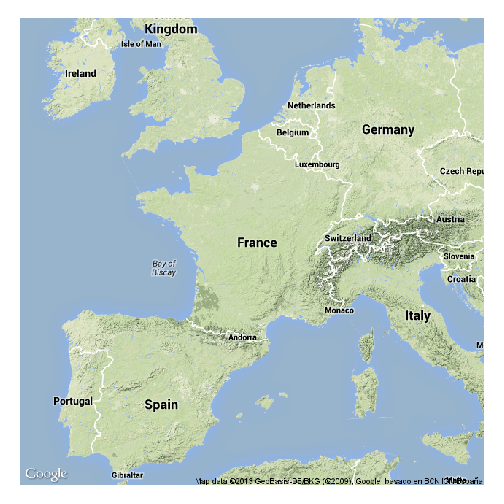Choose map type:

``````mymap <- gmap("France", type = "satellite")
plot(mymap)
``````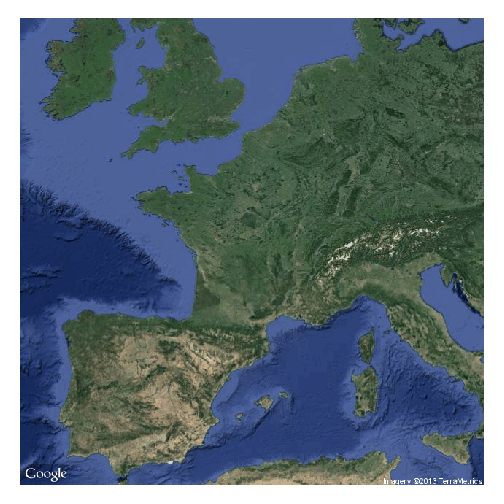Choose zoom level:

``````mymap <- gmap("France", type = "satellite", exp = 3)
plot(mymap)
``````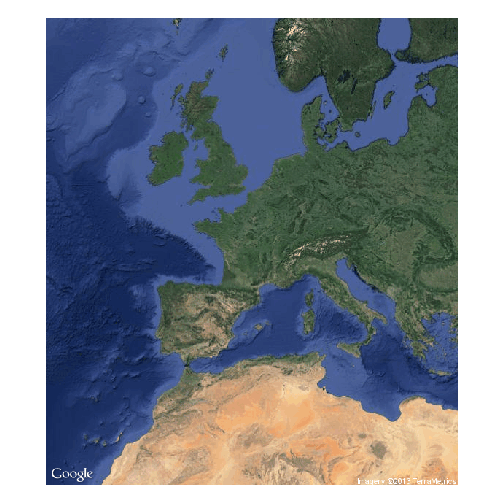Save the map as a file in your working directory for future use

``````mymap <- gmap("France", type = "satellite", filename = "France.gmap")
``````

Now get a map for a region drawn at hand

``````
mymap <- gmap("Europe")
plot(mymap)

select.area <- drawExtent()
# now click 2 times on the map to select your region
mymap <- gmap(select.area)
plot(mymap)
# See ?gmap for many other possibilities
``````

## `RgoogleMaps`: Map your data onto Google Map tiles

``````library(RgoogleMaps)
``````

Get base maps from Google (a file will be saved in your working directory)

``````newmap <- GetMap(center = c(36.7, -5.9), zoom = 10, destfile = "newmap.png",
maptype = "satellite")

# Now using bounding box instead of center coordinates:
newmap2 <- GetMap.bbox(lonR = c(-5, -6), latR = c(36, 37), destfile = "newmap2.png",
maptype = "terrain")

# Try different maptypes
newmap3 <- GetMap.bbox(lonR = c(-5, -6), latR = c(36, 37), destfile = "newmap3.png",
maptype = "satellite")
``````

Now plot data onto these maps, e.g. these 3 points

``````PlotOnStaticMap(lat = c(36.3, 35.8, 36.4), lon = c(-5.5, -5.6, -5.8), zoom = 10,
cex = 4, pch = 19, col = "red", FUN = points, add = F)
``````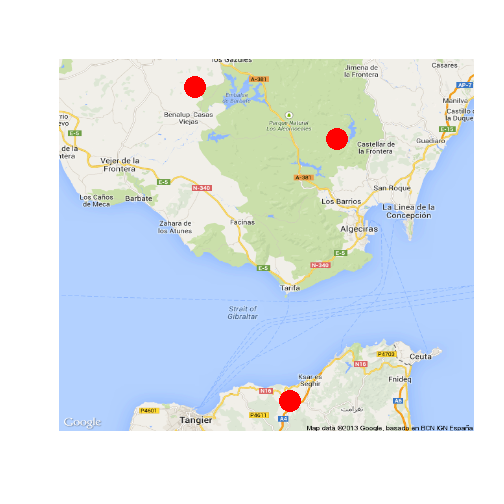## `googleVis`: visualise data in a web browser using Google Visualisation API

``````library(googleVis)
``````

Run `demo(googleVis)` to see all the possibilities

### Example: plot country-level data

``````data(Exports)    # a simple data frame
Geo <- gvisGeoMap(Exports, locationvar="Country", numvar="Profit",
options=list(height=400, dataMode='regions'))
plot(Geo)
``````

Using `print(Geo)` we can get the HTML code to embed the map in a web page!

### Example: Plotting point data onto a google map (internet)

``````data(Andrew)
M1 <- gvisMap(Andrew, "LatLong", "Tip",
options=list(showTip=TRUE, showLine=F, enableScrollWheel=TRUE,
mapType='satellite', useMapTypeControl=TRUE, width=800,height=400))
plot(M1)
``````

## `RWorldMap`: mapping global data

Some examples

``````
library(rworldmap)

newmap <- getMap(resolution = "coarse")  # different resolutions available
plot(newmap)
``````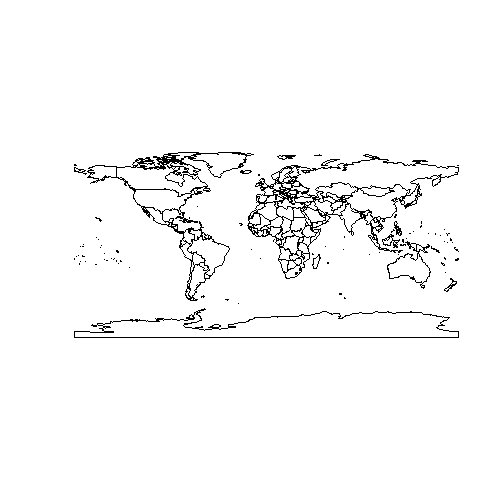``````mapCountryData()
``````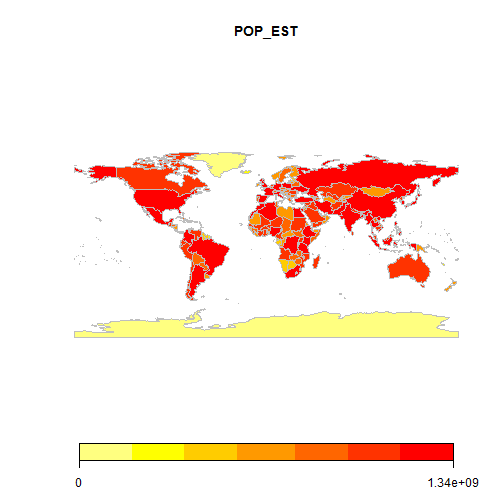``````mapCountryData(mapRegion = "europe")
``````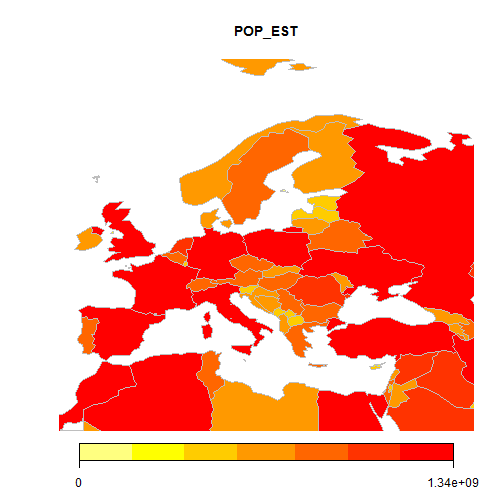``````mapGriddedData()
``````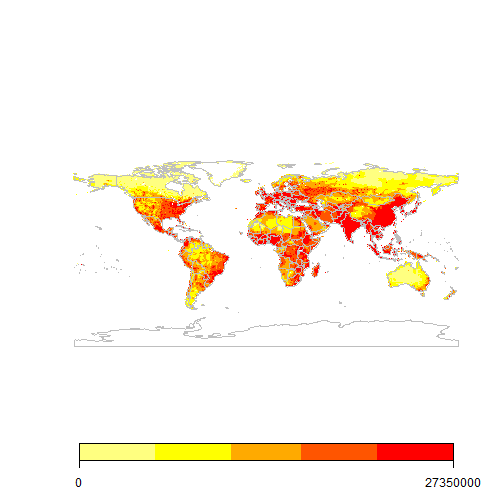``````mapGriddedData(mapRegion = "europe")
``````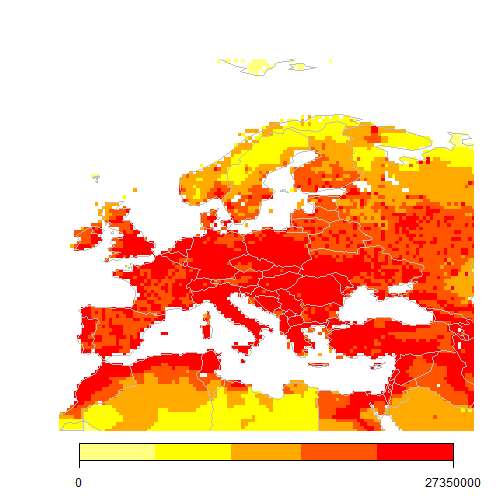# 3. SPATIAL VECTOR DATA (points, lines, polygons)

### Example dataset: retrieve point occurrence data from GBIF

Let's create an example dataset: retrieve occurrence data for the laurel tree (Laurus nobilis) from the Global Biodiversity Information Facility (GBIF)

``````library(dismo)  # check also the nice 'rgbif' package!
laurus <- gbif("Laurus", "nobilis")
``````
``````## Laurus nobilis : 2120 occurrences found
## 1-1000-2000-2120
``````
``````# get data frame with spatial coordinates (points)
locs <- subset(laurus, select = c("country", "lat", "lon"))
head(locs)  # a simple data frame with coordinates
``````
``````##   country   lat    lon
## 1   Spain 36.12 -5.579
## 2   Spain 38.26 -5.207
## 3   Spain 36.11 -5.534
## 4   Spain 36.87 -5.312
## 5   Spain 37.30 -1.918
## 6   Spain 36.10 -5.545
``````
``````
# Discard data with errors in coordinates:
locs <- subset(locs, locs\$lat < 90)
``````

### Making data 'spatial'

So we have got a simple dataframe containing spatial coordinates. Let's make these data explicitly spatial

``````coordinates(locs) <- c("lon", "lat")  # set spatial coordinates
plot(locs)
``````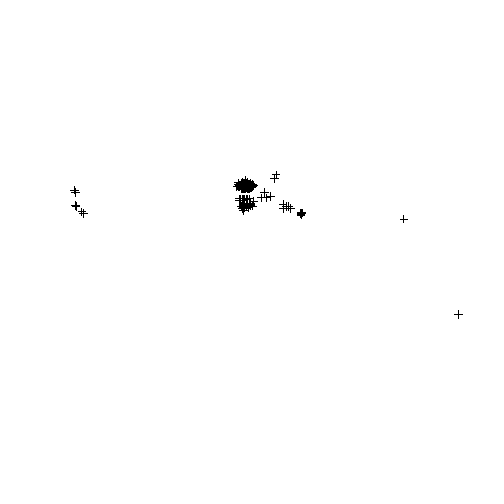### Define spatial projection

Important: define geographical projection. Consult the appropriate PROJ.4 description here: http://www.spatialreference.org/

``````crs.geo <- CRS("+proj=longlat +ellps=WGS84 +datum=WGS84")  # geographical, datum WGS84
proj4string(locs) <- crs.geo  # define projection system of our data
summary(locs)
``````
``````## Object of class SpatialPointsDataFrame
## Coordinates:
##         min    max
## lon -123.25 145.04
## lat  -37.78  59.84
## Is projected: FALSE
## proj4string :
## [+proj=longlat +ellps=WGS84 +datum=WGS84 +towgs84=0,0,0]
## Number of points: 2109
## Data attributes:
##    Length     Class      Mode
##      2109 character character
``````

### Quickly plotting point data on a map

``````plot(locs, pch = 20, col = "steelblue")
library(rworldmap)
# library rworldmap provides different types of global maps, e.g:
data(coastsCoarse)
data(countriesLow)
``````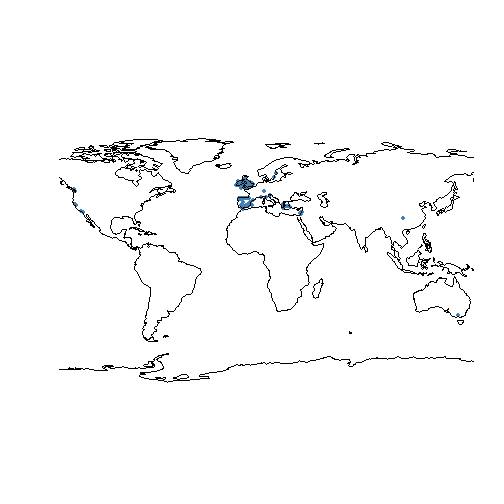### Subsetting and mapping again

``````table(locs\$country)  # see localities of Laurus nobilis by country
``````
``````##
##      Australia         Canada        Croatia         France        Germany
##              2              1              1              1              1
##         Greece        Ireland         Israel          Italy          Spain
##              5             69           1231              2            206
##         Sweden United Kingdom  United States
##              2            578             10
``````
``````
locs.gb <- subset(locs, locs\$country == "United Kingdom")  # select only locs in UK
plot(locs.gb, pch = 20, cex = 2, col = "steelblue")
title("Laurus nobilis occurrences in UK")
``````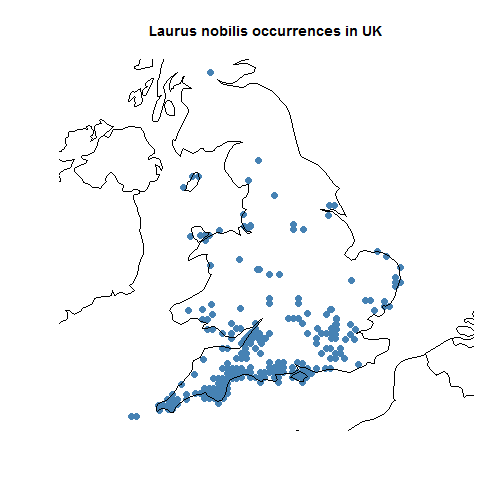``````summary(locs.gb)
``````
``````## Object of class SpatialPointsDataFrame
## Coordinates:
##        min    max
## lon -6.392  1.772
## lat 49.951 56.221
## Is projected: FALSE
## proj4string :
## [+proj=longlat +ellps=WGS84 +datum=WGS84 +towgs84=0,0,0]
## Number of points: 578
## Data attributes:
##    Length     Class      Mode
##       578 character character
``````

## Mapping vectorial data (points, polygons, polylines)

### Mapping vectorial data using `gmap` from `dismo`

``````gbmap <- gmap(locs.gb, type = "satellite")
locs.gb.merc <- Mercator(locs.gb)  # Google Maps are in Mercator projection.
# This function projects the points to that projection to enable mapping
plot(gbmap)
````````````points(locs.gb.merc, pch = 20, col = "red")
``````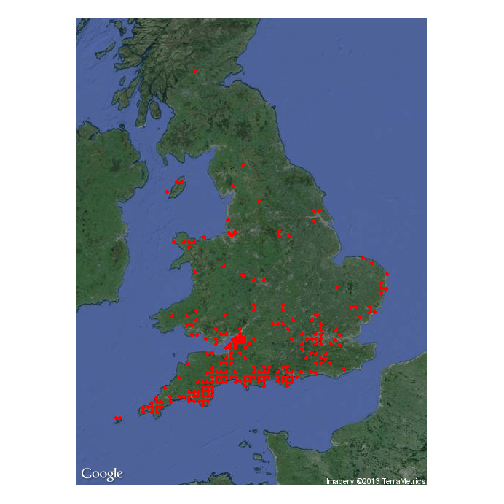### Mapping vectorial data with `RgoogleMaps`

``````